# Math Formulas You Should Memorize

09/21/2015

## Math Help: Useful Formulas

### Probability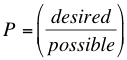The probability (P) of an event occurring is measured as a fraction (between 0 and 1) with 1 being 100% probability and 0 being 0%. Take the number of desired outcomes and divide by the number of possible outcomes. For example, if you want to know the probability of rolling a 3 on a six-sided di, it is 1 (because there is only one 3) over 6 (because there are 6 possibilities). To find the probability two, mutually exclusive events both occurring, multiply their probabilities. If you want to know the probability of rolling a 3 and then a 4, you would multiply 1/6*1/6 for 1/36.

### Midpoint Formula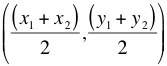When you need to find the midpoint of a line, use this formula to determine the coordinates.

### Distance Formula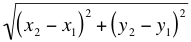If you know your Pythagorean theorem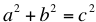, this equation will be intuitive. To determine the distance between two points, you can make a right triangle from them. square each side and then take the square root of the sum to obtain the length of the hypotenuse.

### Cylinder Volume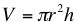The volume of a cylinder is the area of the circle that makes up its end times the height of the cylinder.

### Average Speed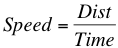To find the average speed, use the formula distance over time.

## For Tutoring in Houston

The Tutoring Center in Houston has the one-to-one tutoring program you need to excel in your academic career. Whether you are struggling, need to refresh, or wish to get ahead, we have a program for you. Check out our academic programs in math, reading, writing, and more on our website. Then call 832-571-8224 to schedule your free diagnostic assessment.

### FREE CONSULTATION

Schedule your Free Diagnostic Assessment Today!